## Friday, July 8, 2016

### More user control in `plot` method for `phylo.toBackbone` (objects of class `"backbonePhylo"`)

I just pushed an update to the S3 `plot` method for objects of class `"backbonePhylo"` that allows for better user control of the plotted triangles.

The idea is that sometimes we may want to plot the triangles of the collapsed subtrees to have a fixed height (this is now permitted with the argument `fixed.height=TRUE`), and/or we may want to show the diversity of the collapsed subtree (`print.clade.size=TRUE`). These two options are off by default, as is the option `fixed.n1`, which tells `plot.backbonePhylo` what to do with the height of clades containing only one taxon when `fixed.height=TRUE`. If `FALSE`, the default, it will plot as a leaf, not a clade.

For instance:

``````library(phytools)
``````
``````## Loading required package: ape
``````
``````## Loading required package: maps
``````
``````##
##  # maps v3.1: updated 'world': all lakes moved to separate new #
##  # 'lakes' database. Type '?world' or 'news(package="maps")'.  #
``````
``````packageVersion("phytools")
``````
``````##  '0.5.40'
``````
``````## object of class "backbonePhylo"
obj
``````
``````##
## Backbone phylogenetic tree with 11 subtrees and 10 resolved internal nodes.
##
## Labels: t10, t2, A, B, C, ...
## Diversities: 1, 1, 8, 17, 19, ...
``````
``````## the default
plot(obj)
``````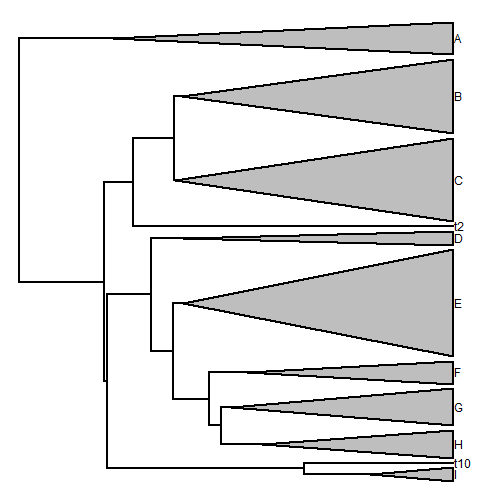``````## fixed heights
plot(obj,fixed.height=TRUE)
``````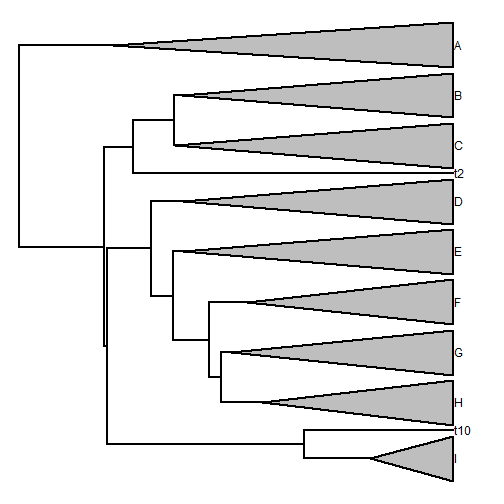``````## show clade sizes
``````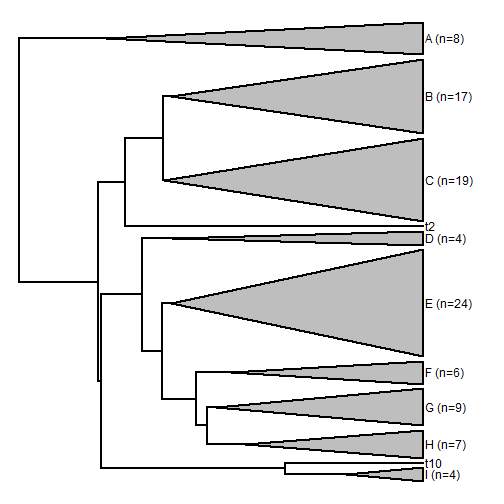``````## both
``````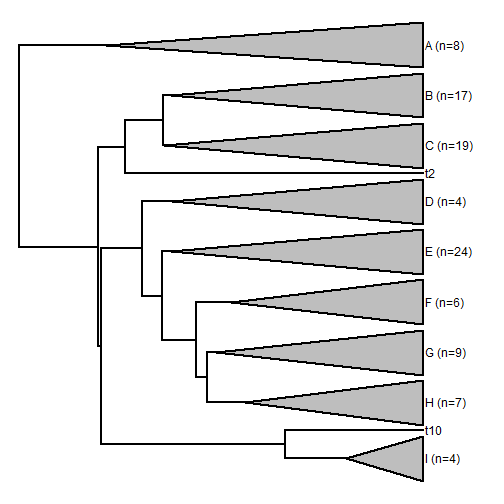``````## both, plus fixed.n1=TRUE
fixed.n1=TRUE)
``````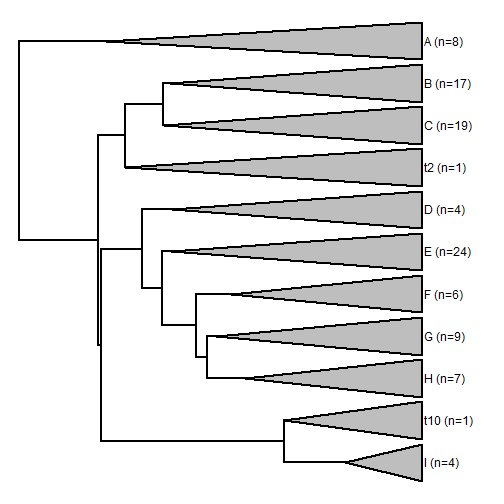I also added the argument `sep` which sets the clade separation, in units of the vertical dimension of the plotting device - which goes from zero through the number of tips in the uncollapsed tree (or the sum of the diversity of each subtree). This is true regardless of whether or not the height of each subtree is standardized. E.g.:

``````plot(obj,fixed.height=TRUE,print.clade.size=TRUE,sep=2)
``````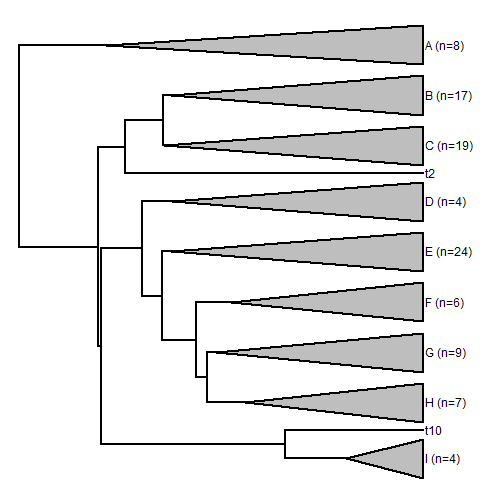``````plot(obj,fixed.height=TRUE,print.clade.size=TRUE,sep=0.4)
``````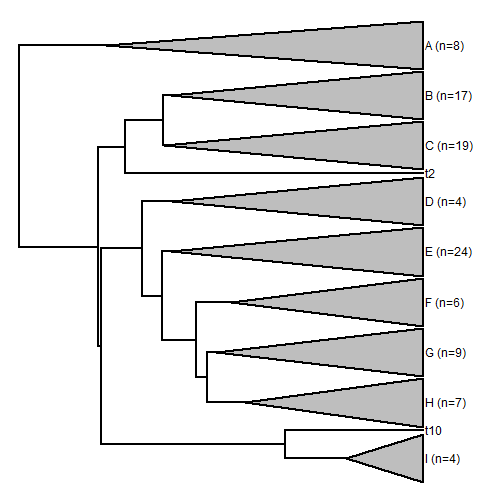Remember, we can also use colors, e.g.:

``````library(RColorBrewer)
colors<-setNames(brewer.pal(Ntip(obj),"Paired"),
colors
``````
``````##       t10        t2         A         B         C         D         E
## "#A6CEE3" "#1F78B4" "#B2DF8A" "#33A02C" "#FB9A99" "#E31A1C" "#FDBF6F"
##         F         G         H         I
## "#FF7F00" "#CAB2D6" "#6A3D9A" "#FFFF99"
``````
``````plot(obj,fixed.height=TRUE,print.clade.size=TRUE,
col=colors)
``````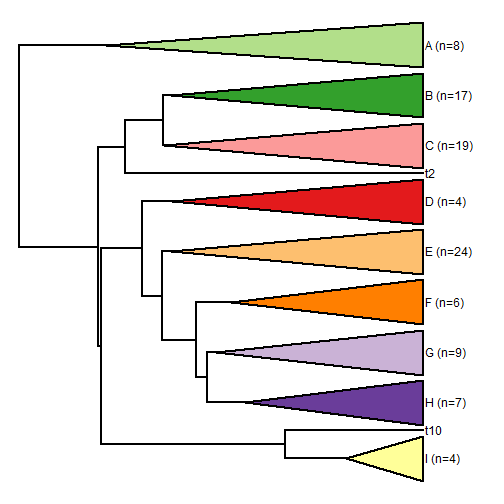As always, the aliasing is due to the plotting device & we can easily fix it by plotting to PDF (or other vector format, depending on our machine):

``````pdf(file="plot.backbonePhylo.pdf")
col=colors)
dev.off()
``````
``````## png
##   2
``````

See plot plot.backbonePhylo.pdf

That's it.